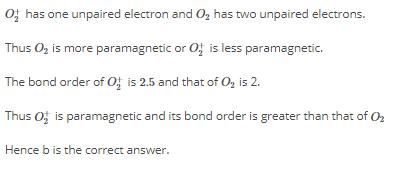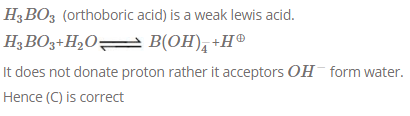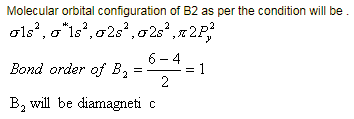Courses

# MCQ (Previous Year Questions) - Chemical Bonding & Molecular Structure (Level 2)

## 25 Questions MCQ Test Chemistry for JEE Advanced | MCQ (Previous Year Questions) - Chemical Bonding & Molecular Structure (Level 2)

Description
This mock test of MCQ (Previous Year Questions) - Chemical Bonding & Molecular Structure (Level 2) for JEE helps you for every JEE entrance exam. This contains 25 Multiple Choice Questions for JEE MCQ (Previous Year Questions) - Chemical Bonding & Molecular Structure (Level 2) (mcq) to study with solutions a complete question bank. The solved questions answers in this MCQ (Previous Year Questions) - Chemical Bonding & Molecular Structure (Level 2) quiz give you a good mix of easy questions and tough questions. JEE students definitely take this MCQ (Previous Year Questions) - Chemical Bonding & Molecular Structure (Level 2) exercise for a better result in the exam. You can find other MCQ (Previous Year Questions) - Chemical Bonding & Molecular Structure (Level 2) extra questions, long questions & short questions for JEE on EduRev as well by searching above.
QUESTION: 1

Solution:
QUESTION: 2

Solution:
QUESTION: 3

### Identify the least stable ion amongst the following                      [JEE 2002]

Solution:
QUESTION: 4

Which of the following molecular species has unpaired electron (s) ?                     [JEE 2002]

Solution:
QUESTION: 5

Which of the following are isoelectronic and isostructural ? NO3_, CO32_, ClO3_, SO[JEE 2003]

Solution:
QUESTION: 6

According to molecular orbital theory which of the following statement about the magnetic character and bond order is correct regarding O2+               [JEE 2004]

Solution:QUESTION: 7

Which species has the maximum number of lone pair of electrons on the central atom ? [JEE 2005]

Solution:
QUESTION: 8

The percentage of p-character is the orbitals forming P_P bonds in P4 is [JEE 2007]

Solution: Hybridisation around P is sp3 (1 lone pair , 3 sigma bond ) so P character is (3×100/4) 75%.
QUESTION: 9

Among the following, the paramagnetic compound is                         [JEE 2007]

Solution: KO2 is a superoxide in which, only one electron is released from the dioxygen atom and a superoxide ion is represented as 02 . So, in KO2 the oxygen atoms bear -1/2 oxidation state and they also behave as a free radical species, having an unpaired electron. We know that a molecule becomes paramagnetic due to the presence of unpaired electrons. Hence, KO2 behaves as paramagnetic molecule.
QUESTION: 10

The species having bond order different from that in CO is                       [JEE 2007]

Solution:
QUESTION: 11

The structure of XeO3 is                            [JEE 2007]

Solution:
QUESTION: 12

Statement-1 : p-Hydroxybenzoic acid has a lower boiling point than o-hydroxybenzoic acid.

Statement-2 : o-Hydroxybenzoic acid has intramolecular hydrogen bonding. [JEE 2007]

Solution:
QUESTION: 13

Statement-1 : In water, orthoboric acid behaves as a weak monobasic acid.

Statement-2 : In water, orthoboric acid acts as a proton donor. [JEE 2007]

Solution:QUESTION: 14

Statement-1 : Pb4+ compounds are stronger oxidizing agents than Sn4+compounds. [JEE 2008]

Statement-2 : The higher oxidation states of group 14 elements are more stable for the heavier members of the group due to 'inert pair effect'.

Solution:
QUESTION: 15

Match each of the diatomic molecules in Column - I with its property/ properties in Column-II.

Column-I Column-II [JEE 2009]

(A) B2 (P) Paramagnetic

(B) N2 (Q) Undergoes oxidation

(C) O2 (R) Undergoes reduction

(D) O2 (S) Bond order ³ 2

(T) Mixing of 's' and 'p' orbitals

Solution:
*Multiple options can be correct
QUESTION: 16

The nitrogen oxide(s) that contain(s) N_N bond(s) is (are).                                                            [JEE 2009]

Solution:
*Multiple options can be correct
QUESTION: 17

In the reaction                                                                                                [JEE 2009]

2X + B2H6 ® [BH2(X)2]+[BH 4]_ the amine(s) X is (are) :

Solution:
*Multiple options can be correct
QUESTION: 18

The species having pyramidal shape is                                                                                           [JEE 2010]

Solution:
*Multiple options can be correct
QUESTION: 19

Assuming that Hund's rule is violated, the bond order and magnetic nature of the diatomic molecule B2 is [JEE 2010]

Solution:QUESTION: 20

All the compounds listed in Column I react with water. Match the result of the respective reactions with the appropriate options listed in Column II.

Column-I Column-II                                                                                                                             [JEE 2010]

(A) (CH3)2SiCl(P) Hydrogen halide formation

(B) XeF(Q) Redox reaction

(C) Cl(R) Reacts with glass

(D) VCl(S) Polymerization

(T) O2 formation

Solution:
*Multiple options can be correct
QUESTION: 21

In Allen (C3H4), the type (s) of hybridisation of the carbon atoms is (are)                                         [JEE 2012]

Solution:
*Multiple options can be correct
QUESTION: 22

The shape of XeO2F2 molecule is                                                                                                    [JEE 2012]

Solution:
*Multiple options can be correct
QUESTION: 23

With respect to graphite and diamond, which of the statement(s) given below is (are) correct?

Solution:
QUESTION: 24

Decreasing order of the O_O bond length present in them O2, KO2 and O2[AsF4]                                                  [JEE 2004]

Solution:
QUESTION: 25

The value of n in the molecular formula BenAl2Si6O18 is [JEE 2010]

Solution: